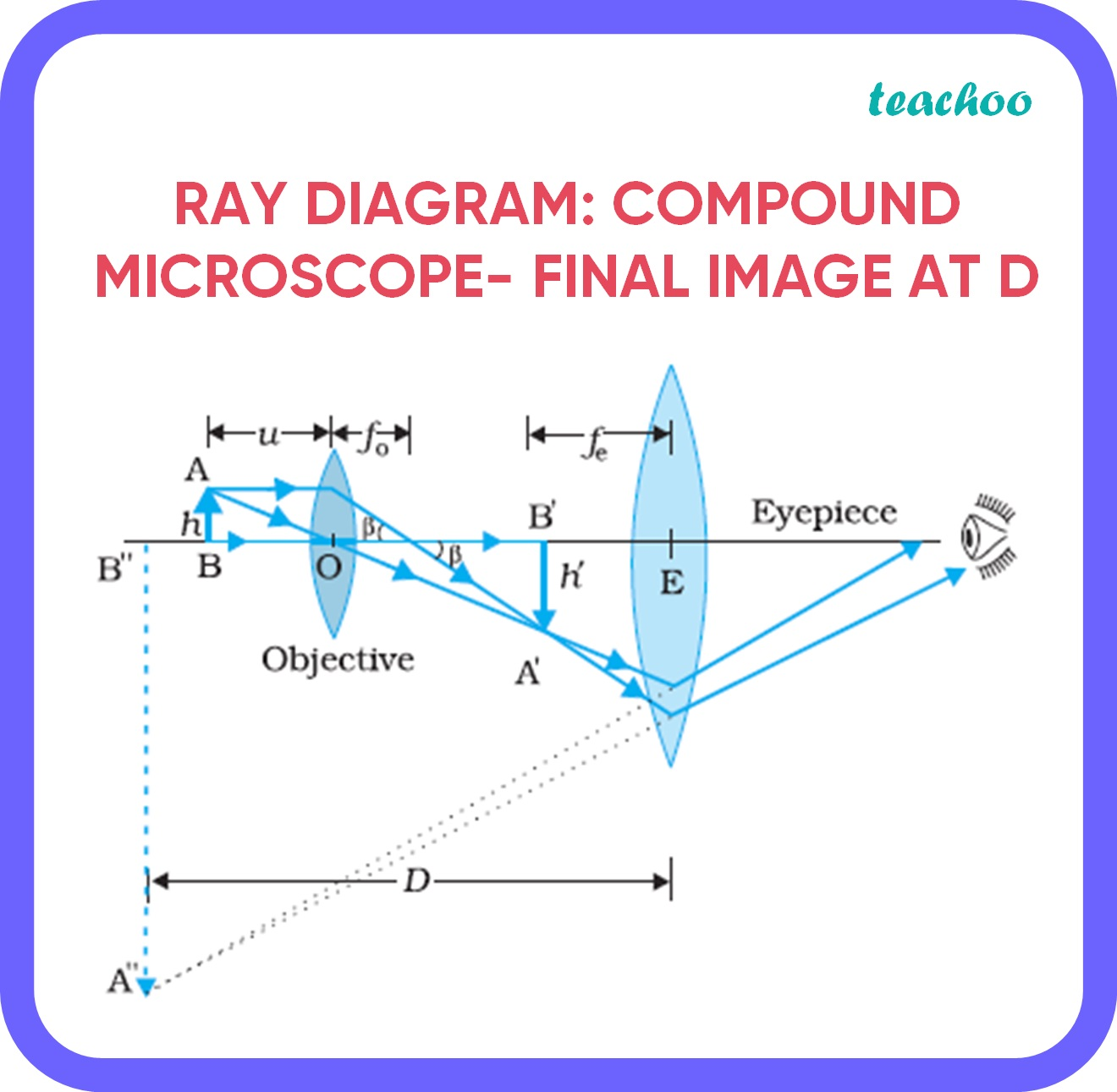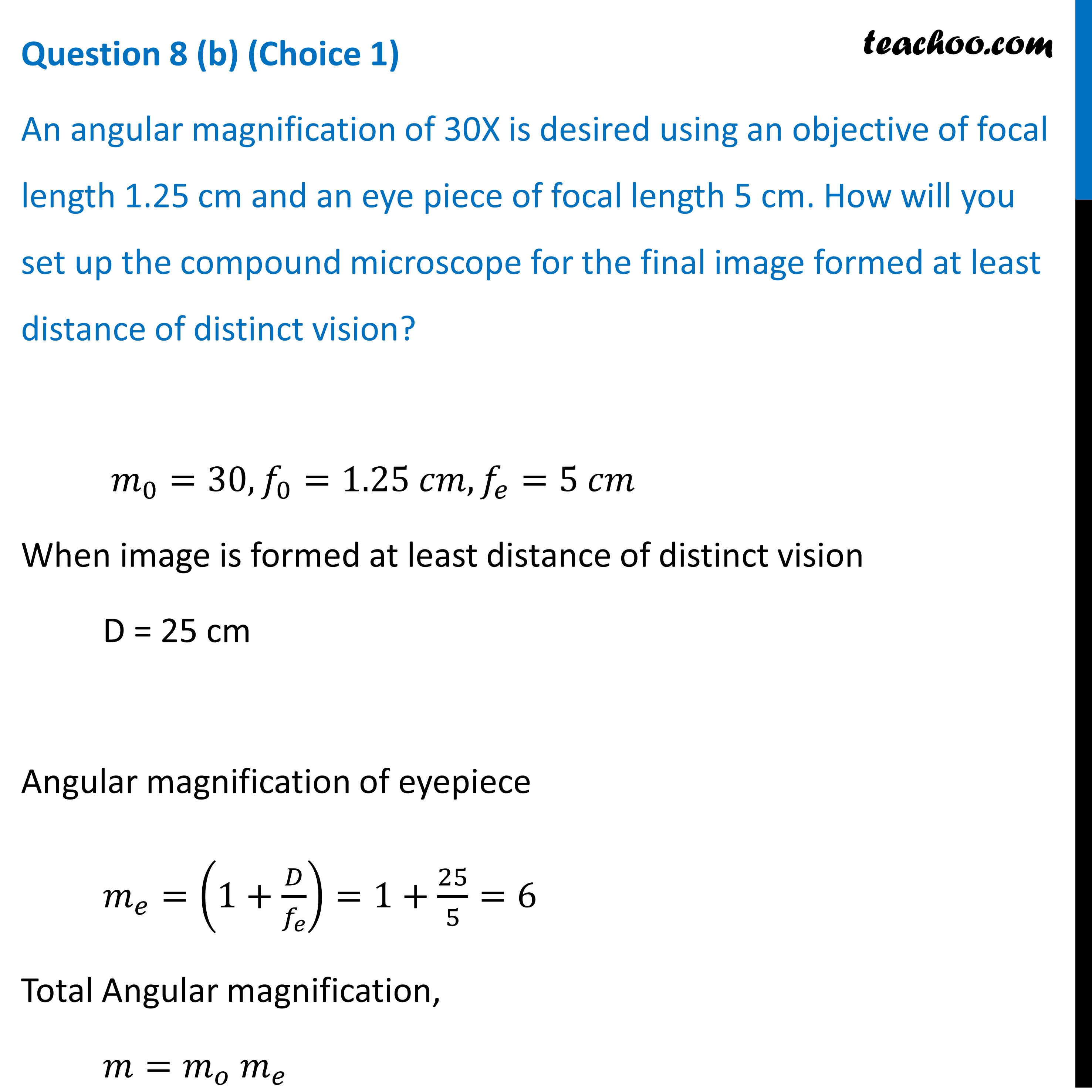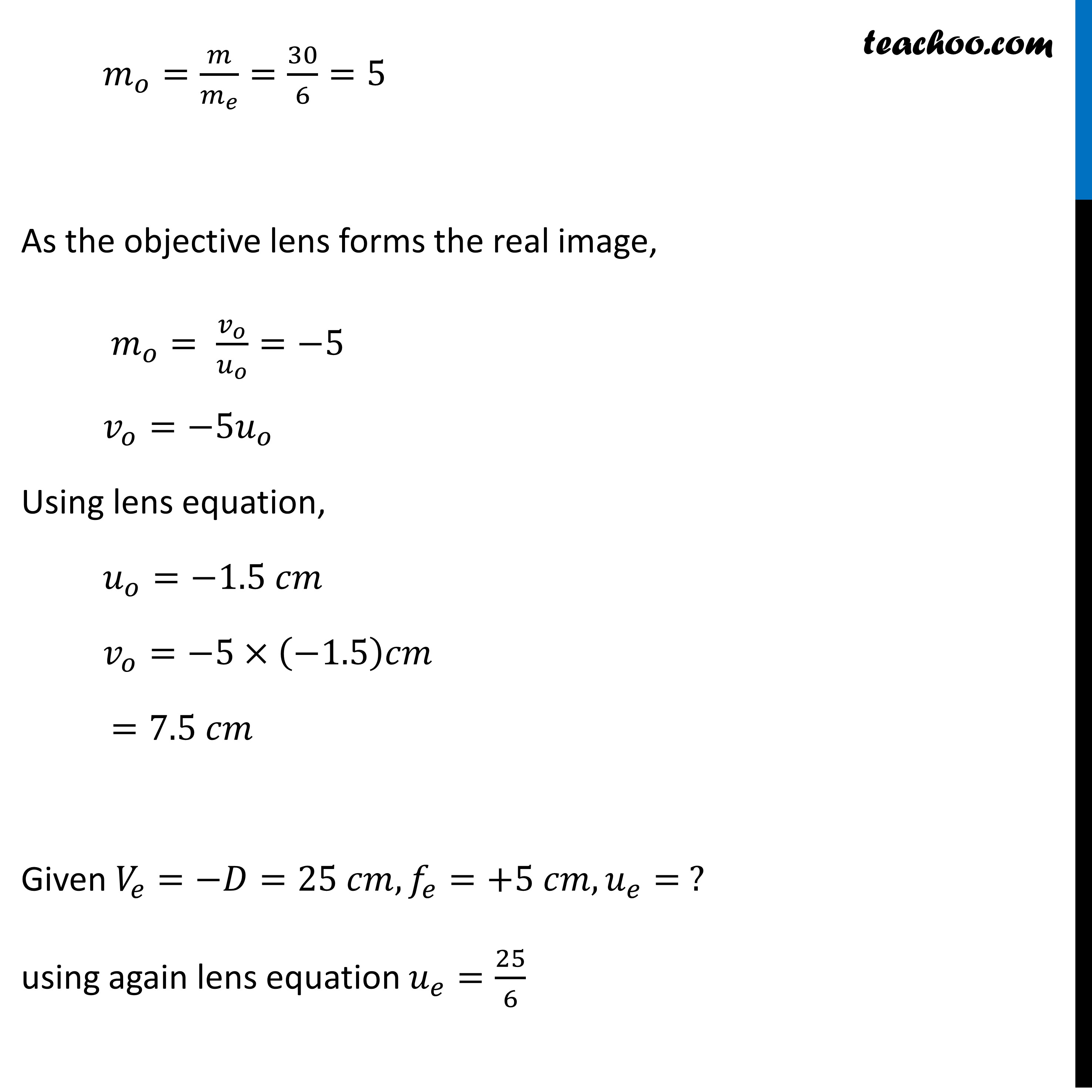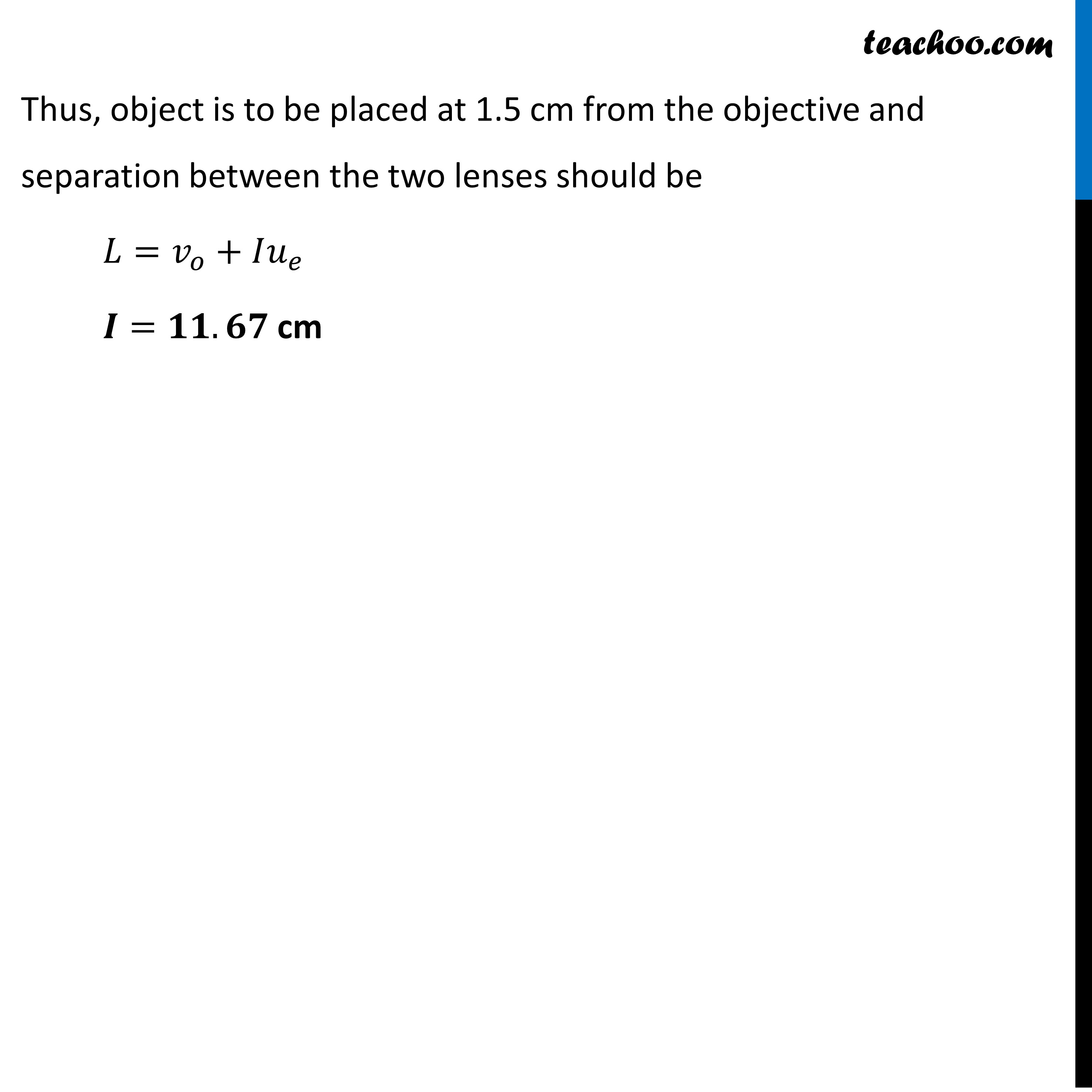CBSE Class 12 Sample Paper for 2022 Boards [Term 2] - Physics

Physics Class 12
Solutions to CBSE Sample Paper - Physics Class 12

## (a) Draw a ray diagram of a compound microscope for the final image formed at least distance of distinct vision?

Diagram of Compound Microscope for the final image formed at D## (b) An angular magnification of 30X is desired using an objective of focal length 1.25 cm and an eye piece of focal length 5 cm. How will you set up the compound microscope for the final image formed at least distance of distinct vision?Learn in your speed, with individual attention - Teachoo Maths 1-on-1 Class

### Transcript

Question 8 (b) (Choice 1) An angular magnification of 30X is desired using an objective of focal length 1.25 cm and an eye piece of focal length 5 cm. How will you set up the compound microscope for the final image formed at least distance of distinct vision? 𝑚_0=30,𝑓_0=1.25 𝑐𝑚,𝑓_𝑒=5 𝑐𝑚 When image is formed at least distance of distinct vision D = 25 cm Angular magnification of eyepiece 𝑚_𝑒=(1+𝐷/𝑓_𝑒 )=1+25/5=6 Total Angular magnification, 𝑚=𝑚_𝑜 𝑚_𝑒 𝑚_𝑜=𝑚/𝑚_𝑒 =30/6=5 As the objective lens forms the real image, 𝑚_𝑜= 𝑣_𝑜/𝑢_𝑜 =−5 𝑣_𝑜=−5𝑢_𝑜 Using lens equation, 𝑢_𝑜=−1.5 𝑐𝑚 𝑣_𝑜=−5×(−1.5)𝑐𝑚 =7.5 𝑐𝑚 Given 𝑉_𝑒=−𝐷=25 𝑐𝑚, 𝑓_𝑒=+5 𝑐𝑚, 𝑢_𝑒= ? using again lens equation 𝑢_𝑒=25/6 Thus, object is to be placed at 1.5 cm from the objective and separation between the two lenses should be 𝐿=𝑣_𝑜+𝐼𝑢_𝑒 𝑰=𝟏𝟏.𝟔𝟕 cm